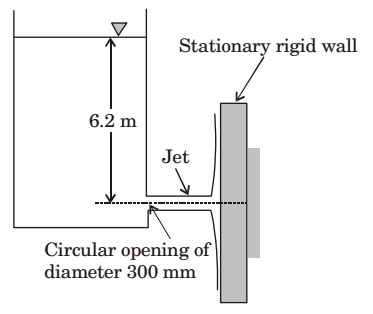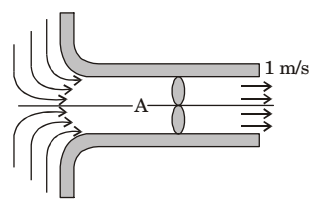## Fluid Mechanics and Hydraulic Machinery Miscellaneous

#### Fluid Mechanics and Hydraulic Machinery

1. The water jet exiting from a stationary tank through a circular opening of diameter 300 mm impinges on a rigid wall as shown in the figure. Neglect all minor losses and assume the water level in the tank to remain constant. The net horizontal force experienced by the wall is ____ kN. Density of water is 1000 kg/m3. Acceleration due to gravity g = 10 m/s².1. Force exerted by a jet of water striking fixed wall = ρav²

 = 1000 × π × 0.3² × V² 4

 = 1000 × π × 0.3² × (2 × 10 × 6²) [∵ V = √2gh] 4

= 8.76 N

##### Correct Option: A

Force exerted by a jet of water striking fixed wall = ρav²

 = 1000 × π × 0.3² × V² 4

 = 1000 × π × 0.3² × (2 × 10 × 6²) [∵ V = √2gh] 4

= 8.76 N

1. Water is coming out from a tap and a falls vertically downwards. At the tap opening, the stream diameter is 20 mm with uniform velocity of 2 m/s. Acceleration due to gravity is 9.81 m/ s². Assuming steady, inviscid flow, constant atmospheric pressure everywhere and neglecting curvature and surface tension effects, the diameter in mm of the stream 0.5 m below the tap is approximately

1. Q = A1 V1 =A2 V2

 ⇒ π (20)² × 2 = π × d² × √2 x 9.8 x 0.5 4 4

d ≈ 15mm

##### Correct Option: B

Q = A1 V1 =A2 V2

 ⇒ π (20)² × 2 = π × d² × √2 x 9.8 x 0.5 4 4

d ≈ 15mm

1. A water container is kept on a weighing balance. Water from a tap is falling vertically into the container with a volume flow rate of Q; the velocity of the water when it hits the water surface is U. At a particular instant of time the total mass of the container and water is m. The force registered by the weighing balance at this instant of time is

1. Volume flow rate = Q
Mass of water strike = ρQ
Velocity of the water when it hit the water surface = U
Force on weighing balance due to water strike = Initial momentum – final momentum
= ρQU – 0= ρQU
(since final velocity is perpendicular to initial velocity)
Now total force on weighing balance = mg + ρQU

##### Correct Option: A

Volume flow rate = Q
Mass of water strike = ρQ
Velocity of the water when it hit the water surface = U
Force on weighing balance due to water strike = Initial momentum – final momentum
= ρQU – 0= ρQU
(since final velocity is perpendicular to initial velocity)
Now total force on weighing balance = mg + ρQU

1. A fan in the duct shown below sucks air from the ambient and expels it as a jet at 1 m/s to the ambient. Determine the gauge pressure at the point marked as A. Take the density of air as 1 kg/m3.1. Applying Bernoulli’s equation between sections (i) & A

 P1 = PA + VA² ρg 2g

 PA - P1 = -VA² ρg 2g

P1 = Pambient
∴ PA - P1 = (PA)gauge
 ∴ (PA)gauge == -VA² ρg 2g

 (PA)gauge = -1 × 1² = 0.5N/m² 2

##### Correct Option: A

Applying Bernoulli’s equation between sections (i) & A

 P1 = PA + VA² ρg 2g

 PA - P1 = -VA² ρg 2g

P1 = Pambient
∴ PA - P1 = (PA)gauge
 ∴ (PA)gauge == -VA² ρg 2g

 (PA)gauge = -1 × 1² = 0.5N/m² 2

1. A two-dimensional incompressible frictionless flow field is given by μ = xi - yj. If ρ is the density of the fluid, the expression for pressure gradient vector at any point in the flow field is given as

1. NA

NA